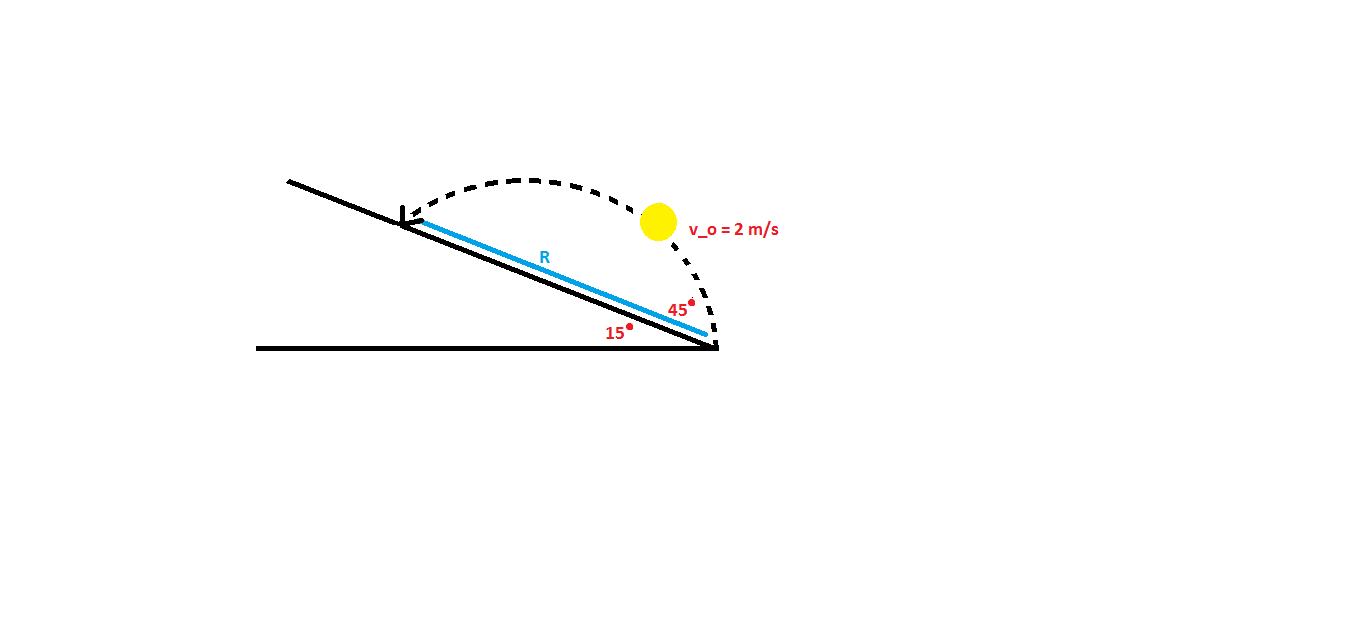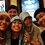# Ball Thrown Up An Inclined PlaneA yellow ball is thrown with initial speed $v_{0} = 2$ $m/s$ up an inclined plane. The plane is inclined at an angle $15 ^ \circ$ above the horizontal, and the ball's thrown with an angle $45 ^ \circ$ above the plane. If $R$ denotes the ball land's distance, find the value of $R$. (assume $g = 10$ $m/s^2$)

I found that the answer is $\frac{2}{5}(\sqrt{3} - 1)$ $m$, but I'm not sure. Could anyone clarify? Thanks :)Note by Nabila Nida Rafida
7 years, 10 months ago

This discussion board is a place to discuss our Daily Challenges and the math and science related to those challenges. Explanations are more than just a solution — they should explain the steps and thinking strategies that you used to obtain the solution. Comments should further the discussion of math and science.

When posting on Brilliant:

• Use the emojis to react to an explanation, whether you're congratulating a job well done , or just really confused .
• Ask specific questions about the challenge or the steps in somebody's explanation. Well-posed questions can add a lot to the discussion, but posting "I don't understand!" doesn't help anyone.
• Try to contribute something new to the discussion, whether it is an extension, generalization or other idea related to the challenge.

MarkdownAppears as
*italics* or _italics_ italics
**bold** or __bold__ bold
- bulleted- list
• bulleted
• list
1. numbered2. list
1. numbered
2. list
Note: you must add a full line of space before and after lists for them to show up correctly
paragraph 1paragraph 2

paragraph 1

paragraph 2

[example link](https://brilliant.org)example link
> This is a quote
This is a quote
    # I indented these lines
# 4 spaces, and now they show
# up as a code block.

print "hello world"
# I indented these lines
# 4 spaces, and now they show
# up as a code block.

print "hello world"
MathAppears as
Remember to wrap math in $$ ... $$ or $ ... $ to ensure proper formatting.
2 \times 3 $2 \times 3$
2^{34} $2^{34}$
a_{i-1} $a_{i-1}$
\frac{2}{3} $\frac{2}{3}$
\sqrt{2} $\sqrt{2}$
\sum_{i=1}^3 $\sum_{i=1}^3$
\sin \theta $\sin \theta$
\boxed{123} $\boxed{123}$

Sort by:

We need to start by finding final speed, $v_f$.

After that, u can start using conservation of mechanical energy

$K_i + U_i = K_f + U_f$

$\frac{1}{2}mv_0{}^{2} = \frac{1}{2} mv_f{}^{2} + mgR\sin 45^{\circ}$

$v_0{}^{2} = v_f{}^{2} + 2gR\sin 45^{\circ}$

Now u have all the values except $R$ and u can easily find $R$

- 7 years, 9 months ago

What is the value of $v_f$?

- 7 years, 9 months ago

U can use the equation of kinematics

In the y-direction

$v_{yf}^{2} = (v_0\sin45^{\circ})^{2} -2gR\sin45^{\circ}$

$v_{yf} = v_0\sin 45^{\circ} - gt$

$R\sin 45^{\circ} = v_0\sin 45^{\circ}t - \frac{1}{2} gt^{2}$

$R\sin 45^{\circ} = \frac{1}{2}(v_{yf} + v_0\sin 45^{\circ})t$

In the x-direction

$R\cos 45^{\circ} = v_0\cos 45^{\circ}t$

Using the equations, we can find $v_{yf}$. We also have $v_{xf} = v_{xi} = v_0\cos 45^{\circ}$. Then u can find $v_f$ by Pythagoras theorem: $v_f = \sqrt{v_{xf}^{2}+v_{yf}^{2}}$

- 7 years, 9 months ago

rotate Oxy 15

gx= -10sin15; gy= -10cos15

t = 2vsin45/gy

R=vcos45t - 1/2 * gx * t^2 =2*(1 - tan15)/cos15

- 7 years, 10 months ago

$Range=\frac{ 2*\sin45 }{ 5 *\cos^215 }$

- 7 years, 10 months ago

i think it would be R=uusin2*/g=0.4m

- 7 years, 10 months ago

tan15=y/x =(2.sin60.t-1/2.10. t^2)/(2.cos60.t)
2-sqrt{3} =sqrt{3}-5t
t={2/5}(sqrt{3}-1)
R= x/sin 15 =t/sin 15 = {4/5}sqrt{2}

- 7 years, 10 months ago

R = x / cos 15

- 7 years, 10 months ago

Yes you are right Lokesh. R=x/cos15 that gives me the same answer as yours

- 7 years, 10 months ago

$\frac{ 4 }{ 5 }(2\sqrt{6}-3\sqrt{2})$ ??

- 7 years, 10 months ago

How did you get the answer?

- 7 years, 10 months ago

yes.I agree

- 7 years, 10 months ago

$v_{x} = 2 \cos 60^\circ = 1$

$v_{y} = 2 \sin 60^\circ = \sqrt{3}$

$x = v_{x}t = t$

$y = v_{y}t - \frac{1}{2}gt^2 = \sqrt{3}t - 5t^2$

Because $\tan 15^\circ = \frac{y}{x},$ then $x = t = y(2 + \sqrt{3})$

By substituting $t = y(2 + \sqrt{3})$ to $y = \sqrt{3}t - 5t^2,$

$y = \sqrt{3}(2 + \sqrt{3})y - 5(2 + \sqrt{3})^2y^2$

$y(5(7 + 4\sqrt{3})y - 2(1 + \sqrt{3})) = 0$

Since $y \neq 0,$

$y = \frac{2(1+\sqrt{3})}{5(7+4\sqrt{3})}$

Because $\sin 15^\circ = \frac{y}{R},$ then $R = \frac{y}{\sin 15^\circ}$

Hence, $R = \frac{4\sqrt{2}(2-\sqrt{3})}{5}$

- 7 years, 10 months ago

Thanks, it seems my answer yet completed

- 7 years, 10 months ago

Fariz nice approach to the question, I completely agree with your answer as I verified it using a different method; which was finding the intercepts of the trajectory of the projectile and the plane.

I found using simple physics formulae that the height is $0.15m$

I then used this to model a formula for the trajectory; $y=ax^{2}+bx$ where b is the initial gradient of the projectile $tan(\pi/3)$,

Using height of $0.15$, $a$ was found to be $-5$

Hence, $y=\sqrt{3}x-5x^{2}$ is the trajectory in the Cartesian coordinate system

This is now intercepted with the plane which is linear; $y=bx$, were b is $tan(\pi/12)$

Therefore the plane is represented as; $y=(2-\sqrt{3})x$

Furthermore, the coordinates of the intercepts of the trajectory and the plane are calculated to be:

$y=\frac{6}{5}(\sqrt{3}-2)$ and $x=\frac{2}{5}(\sqrt{3}-1)$

Finally, using Pythagoras's Theorem, $R=\sqrt{(\frac{2}{5}(\sqrt{3}-1))^{2}+(\frac{6}{5}(\sqrt{3}-2))^{2} }$

Hence, $R=\frac{4}{5}\sqrt{2}(2-\sqrt{3})$

- 7 years, 10 months ago

Nice one!

- 7 years, 10 months ago

Mathematical approach, cool :)

- 7 years, 10 months ago

Nice solution, though just a slight mistake in the end. I believe, you can easily get the right answer if you try to deduce a point in the trajectory where $\frac {y} {x} = \tan 15^\circ$ using the above equations. This would give the required R most probably.

- 7 years, 10 months ago

Would you like to fix it, Lokesh? I'm not sure about my answer.....

- 7 years, 10 months ago

Referring to your solution, equation (3) and equation (4) define the trajectory of ball. The ball lands at the point $(x, y)$ such that $\frac {y} {x} = tan 15^ \circ$.

Solving these three equations, the point can be located and hence the distance where the ball lands. Diving equation (4) with equation (3), $\frac {y} {x} = \sqrt3 - 5t$ and using $\frac {y} {x} = tan 15^ \circ$, finally $\sqrt3 - 5t = tan 15^ \circ \Rightarrow \sqrt3 - 5t = 2 - \sqrt(3)$.

Solving this we have $x = t =\frac {2 \sqrt3 - 2}{5}$. Using trigonometry we get $\frac{x} {R} = cos 15^ \circ$ and hence $R = \frac{ x }{ cos 15^ \circ} \Rightarrow R = \frac {4(2 \sqrt3 - 2)}{5(\sqrt6 + \sqrt2)}$.

Finally, $R = \frac {4(2\sqrt2 - \sqrt 6)}{5}$ which is same as yours. Hahaha. I was wrong about your solution. Sorry about that one.

- 7 years, 10 months ago

Haha it's okay.

- 7 years, 10 months ago

Hey, I think what you found was incorrect in the sense that what you found wasn't what the question was asking for. I found that your answer was what I got for the horizontal distance, not the actually length between the ball land's distance. What I did was find the equation for both lines (the inclined plane and the trajectory) find the intersection which is where you got, and the last bit is apply the Pythagorean theorem to get that length.

- 7 years, 10 months ago

it should be 4 (sqrt2)/5

- 7 years, 10 months ago

Yes Victor is right. You may even use trigonometry sin 15 = (sqrt3-1)/2

- 7 years, 10 months ago